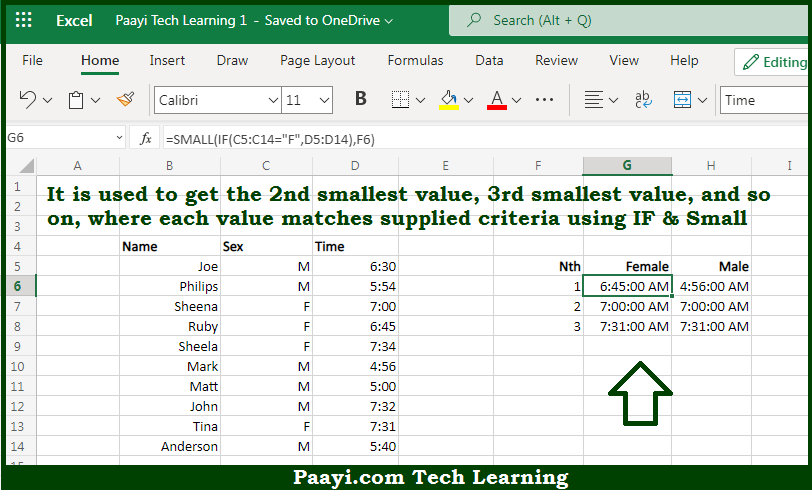# Learn How to Get nth Smallest Value With Criteria in Microsoft Excel

Written by | 0 Comments | 600 Views

In this article, you will learn how to evaluate things with MIN and MAX functions in Microsoft Excel using a single/combination(s) of functions. You will also know to Get nth Smallest Value With Criteria and see the generic formula.

Learn How to Get nth Smallest Value With Criteria in Microsoft Excel

The main purpose of this formula is to get the 2nd smallest value, 3rd smallest value, 4th smallest value, and so on, where each value matches supplied criteria. Here we will learn how to get the nth smallest value with criteria in the given data range in the workbook in Microsoft Excel. That implies, with the help of a formula based on the SMALL and IF functions you can able to get the 2nd smallest value, 3rd smallest value, 4th smallest value, and so on, where each value matches supplied criteria. So, with the help of this formula, you can able to get the nth smallest value with criteria in the given data range in the workbook in Microsoft Excel.

General Formula to Get nth Smallest Value With Criteria

{=SMALL(IF(criteria,values),n)}

The Explanation to Get nth Smallest Value With CriteriaSo we know that with the help of the given formula above you can able to get the 2nd smallest value, 3rd smallest value, 4th smallest value, and so on, where each value matches supplied criteria. Here we will learn how to get the nth smallest value with criteria in the given data range in the workbook in Microsoft Excel. As we know that SMALL function is fully automatic. We just need to feed a data range and an integer for "nth" to specify the ranked value you want. In the example given here, we don't want the SMALL function to operate on every value in the range. We just want values that are either male or female. To perform these criteria, we need the IF function, which provides a logical test for either "M" or 'F". Because we are applying the test to an array of values, the result will also be an array. So now you have learned how to name the nth smallest value with criteria in the given data range in the workbook in Microsoft Excel.